• Make a better intuition about backpropagation
• Technically a little more complicated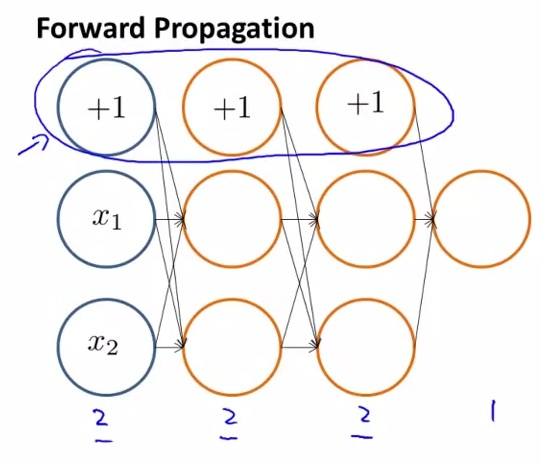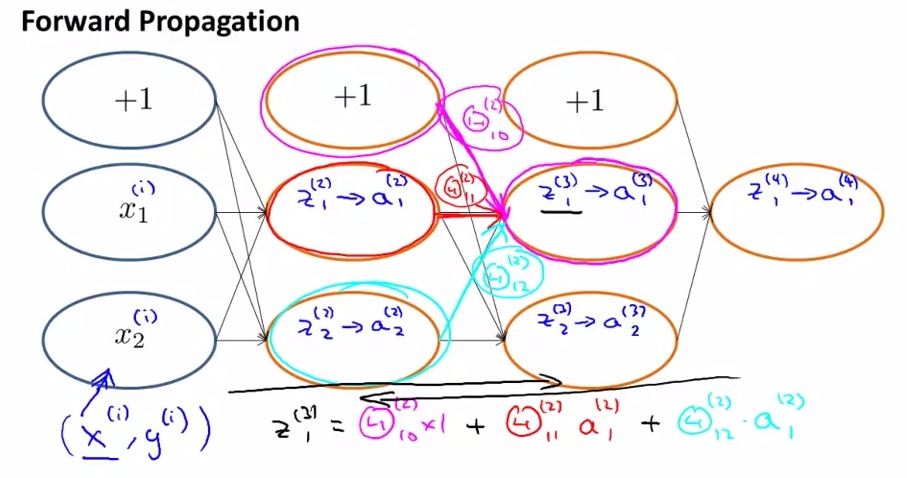• As from example, there's two unit + 1 unit biased unit from forward propagation
• So here's in the example we first take x(1) take it to the neural networks down to the output.
• z(3)1 is the sigmoid function in layer 3 by take summation of (matrix_weight(2)10 x 1(bias_unit) + matrix_weight(2)11 x activation_unit(2)1).
• eventually a(4)1 is the prediction
• Backpropagation is doing the reverse of the forward. The process is really similar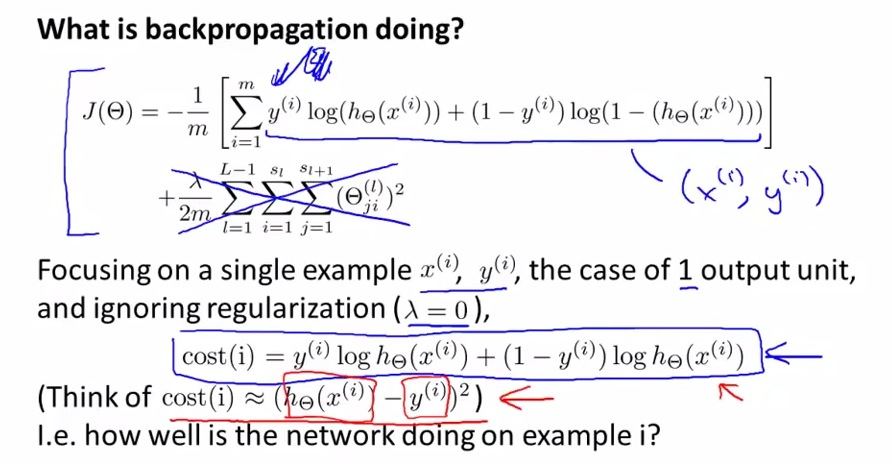• The formula of cost function
• if multiclass, then the formula will be added with summation of K unit classification.
• Because we are doing example of 1 output unit, and 1 example, we are also ignoring the regularization term
• For the purpose of the intuition, log will be ignored. We just want to know how close our network in predicting the output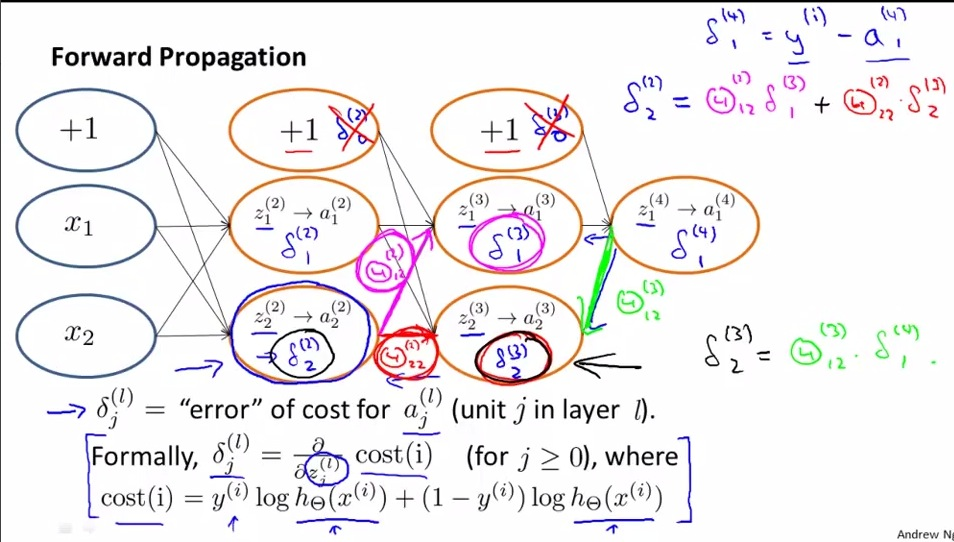• delta can be thought as an error for every activation value
• delta is actually the partial derivative of z, that if we change z, change the cost function, and eventually changing the actual cost
• The first step is intuitive, first final error  = the final actual ouput - the final predicted output
• Then keep going backwards from last layer to first hidden layer
• By going reverse (from right to left) we are acquiring the delta (error value) by calculating [the previous error_value * matrix_weight]
• Layer indexed from 1, the input layer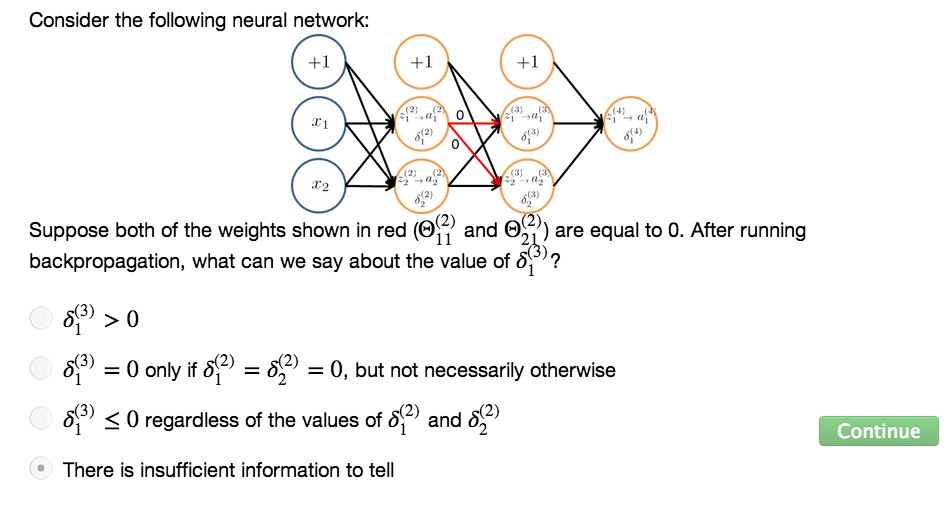• Why? We don't know the value of d(4)1
• Next, give a little better intuition about backpropagation
• Very effective algorithm eventhough a little harder to visualize